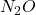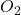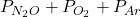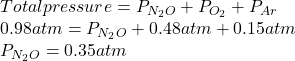## A mixture of oxygen( O2), dinitrogen monoxide (N2O), and argon (Ar) has a total pressure of 0.98 atm. What is the partial pressure of N2O, i

Question

A mixture of oxygen( O2), dinitrogen monoxide (N2O), and argon (Ar) has a total pressure of 0.98 atm. What is the partial pressure of N2O, if the partial pressure of O2 is 0.48 atm and the partial pressure of Ar is 0.15 atm?

in progress 0
7 months 2021-08-01T16:15:22+00:00 1 Answers 8 views 0

1. Answer: The partial pressure ofis 0.35 atm.

Explanation:

Given: Total pressure = 0.98 atm

Partial pressure of= 0.48 atm

Partial pressure of Ar = 0.15 atm

Partial pressure of= ?

Total pressure is the sum of partial pressure of each component present in a mixture of gases.

Hence, partial pressure ofis calculated as follows.

Total pressure =Substitute the values into above formula as follows.Thus, we can conclude that the partial pressure ofis 0.35 atm.